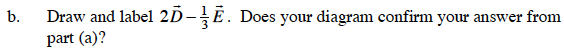### Home > CALC > Chapter 9 > Lesson 9.3.2 > Problem9-103

9-103.

Find vectors $\vec { D }$and $\vec { E }$ on the Lesson 9.3.1 Resource Page.

1. Write $\vec { D }$ and $\vec { E }$ in i, j form. Then find the i, j form for $2 \vec { D } - \frac { 1 } { 3 } \vec { E }$.

2. Draw and label $2 \vec { D } - \frac { 1 } { 3 } \vec { E }$. Does your diagram confirm your answer from part (a)?